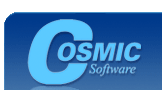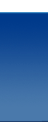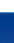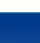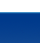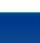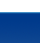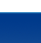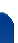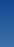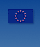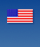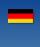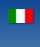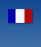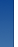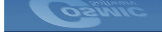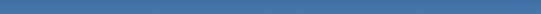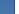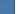Cosmic Software Frequently Asked Questions What order should I link the Cosmic libraries? The cosmic C libraries should be linked next to last in your application link file before the vector table segment (and, anyway, after all application files). The order of libraries themselves depends on the data types used with the libraries. e.g. If your application uses only integer and/or long data types as arguments when calling C library functions then you only need the integer library (libi) and the machine library(libm). ```c:\cosmic\cx__\lib\libi.hXX c:\cosmic\cx__\lib\libm.hXX ``` If your application uses the +sprec compiler option which converts double precision data objects to single precision floating point objects and you use floating point objects as arguments to the C library then you need to use the floating point library(libf), integer library (libi) and the machine library(libm). This ordering also applies to compilers that support only single precision floats such as the ST7, HC05 and HC08 where the +sprec option is not available. ```c:\cosmic\cx__\lib\libf.XXX c:\cosmic\cx__\lib\libi.XXX c:\cosmic\cx__\lib\libm.XXX ``` If you application does NOT uses the +sprec compiler option and uses doubles or floats as arguments when calling C library functions then you need to use the double floating point library(libd), integer library (libi) and the machine library(libm). This is the default ANSI behavior and the safest choice if you're not sure what you need, however it also produces the most code. ```c:\cosmic\cx__\lib\libd.XXX c:\cosmic\cx__\lib\libi.XXX c:\cosmic\cx__\lib\libm.XXX ``` User libraries (that is, any library not provided with the compiler) should be linked just before compiler libaries.